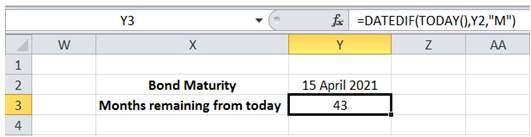## Elapsed number of months in Excel

For example, I want to find the number of months between today and the maturity of a bond (say, 15th Apr 2021).

To do it in Excel, here is the answer:

a) Enter the formula =DATEDIF(TODAY(),Y2,"M") in the cell where the the number of months is required. (Y2 should have the Bond Maturity date).

The TODAY() function returns the current Date. The DATEDIF function subtracts the 2 dates (Bond Maturity Date and Current Date). The use of "M" option returns the number of months between the 2 dates.

Note: Instead of "M", if "Y" is used, number of years is returned. Similarly the use of "D" returns the number of days.